### Problems: Chapter 7

1- Find the shear forces and bending moments at the sections shown. For the bending moment at S3 consider sections right before and after S3.

Section 1 at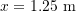: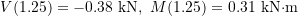.

Section 2 at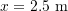: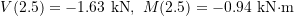.

Section 3 at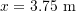: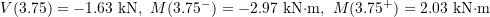.

2- Find the shear forces and bending moments at the sections shown.

Section 1 at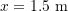: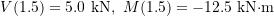.

Section 2 at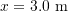: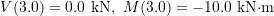.

3- Find the shear forces and bending moments at the sections shown.

Section 1 at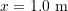: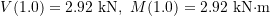.

Section 2 at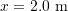: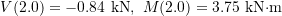.

Section 3 at: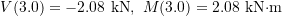.

4- Find the shear forces and bending moments at the sections shown.

Section 1 at: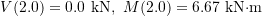.

Section 2 at: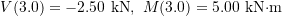.

5- Determines the functions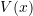and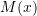.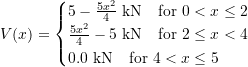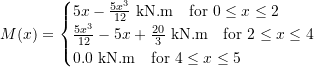6- Determines the functionsand. Consider the support at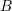as a roller in a slot if the support reaction becomes a pulling force there.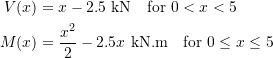7- Determine the functionsand.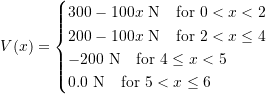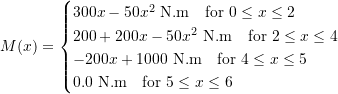8- For the loaded beam shown, find the locations where the shear force and the bending moment attain their maximum magnitudes. Then, determine the maximum magnitudes. Hint: find the local and global extrema (maxima or minima) of the functionsand. Use derivatives for finding local extrema. You can also plotandfor more clarification.

The maximum magnitude of the shear force is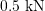and it occurs at two locations being the supports, i.e.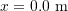and.

The maximum magnitude of the bending moment is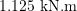occurring at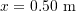.

9- For the loaded beam shown, find the locations where the shear force and the bending moment attain their maximum magnitudes. Then, determine the maximum magnitudes. Hint: see the previous problem.

The maximum magnitude of the shear force is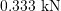occurring at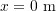, i.e. support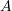.

The maximum magnitude of the bending moment is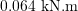occurring at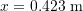.

10- For the loaded beam shown, find the locations where the shear force and the bending moment attain their maximum magnitudes. Then, determine the maximum magnitudes. Hint: see the previous problem.

The maximum magnitude of the shear force isoccurring at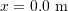and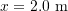i.e. the supports.
The maximum magnitude of the bending moment is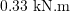occurring at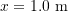.
The maximum magnitude of the shear force is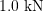occurring ati.e. the support.
The maximum magnitude of the bending moment is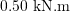occurring ati.e. the support.